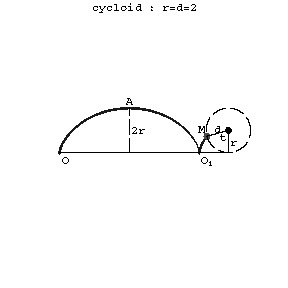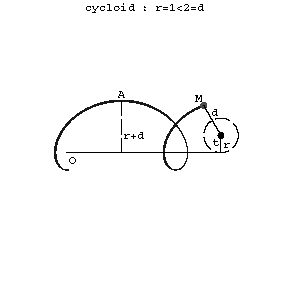# Cycloid

The plane transcendental curve that is the trajectory of a point of a circle rolling along a straight line (Fig. a).Figure: c027540a

The parametric equations are:

$$x=rt-r\sin t,$$

$$y=r-r\cos t,$$

where $r$ is the radius of the circle and $t$ the angle of rotation of the circle. In Cartesian coordinates the equation is:

$$x=r\arccos\frac{r-y}{r}-\sqrt{2ry-y^2}.$$

A cycloid is a periodic curve: the period (basis) is $OO_1=2\pi r$. The points $O,O_k=(2k\pi r,0)$, $k=\pm1,\pm2,\ldots,$ are cusps. The points $A=(\pi r,2r)$ and $A_k=((2k+1)\pi r,2r)$ are the so-called vertices. The area is $S_{OAO_1O}=3\pi r^2$, the radius of curvature is $r_k=4r\sin(t/2)$.

If the curve is described by a point lying outside (inside) a circle rolling along a line, then it is called an extended, (or elongated, or prolate, Fig. b), a contracted, (or shortened, or curtate, Fig. c) cycloid or sometimes a trochoid.Figure: c027540bFigure: c027540c

The parametric equations are

$$x=rt-d\sin t,$$

$$y=r-d\cos t,$$

where $d$ is the distance of the point $M$ from the centre of the rolling circle.

The cycloid is a tautochronic (or isochronic) curve, that is, a curve for which the time of descent of a material point along this curve from a certain height under the action of gravity does not depend on the original position of the point on the curve.